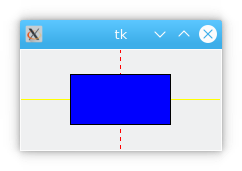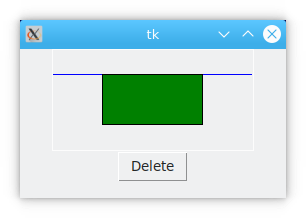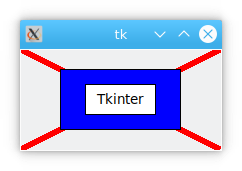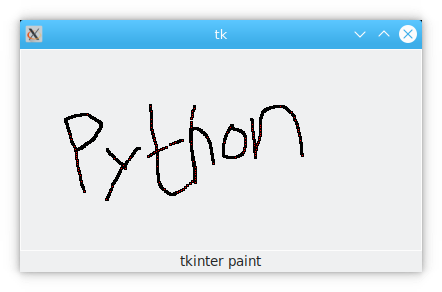# tkinter canvas

The tkinter canvas component provides the basis for Tkinter’s graphical rendering. Highly flexible component that you can use to draw graphs and charts, create graphic editors, and implement a variety of custom widgets.

Canvas is a widget that is commonly used to display and edit graphics. You can use it to draw lines, circles, polygons, and even draw other components.

## tkinter canvas example

To draw an object on the Canvas component, you can use the method create_xxx() (xxx means object type, such as line segment, rectangle, text, etc.).

``````import tkinter as tk
root = tk.Tk()

w = tk.Canvas(root, width =200, height = 100)
w.pack()

# Draw a yellow line across
w.create_line(0, 50, 200, 50, fill = " yellow")
# Draw a red vertical line (dashed line)
w.create_line(100, 0, 100, 100, fill = "red ", dash = (4, 4))
# Draw a blue rectangle in the middle
w.create_rectangle(50, 25, 150, 75, fill = "blue")

root.mainloop()``````Note that the objects added to the Canvas will always be kept. If you wish to modify them, you can use `coords()`, `itemconfig()` and the `move()` method to move an object on the canvas, or `delete()` method to delete.

``````import tkinter as tk

root = tk.Tk()

w = tk.Canvas(root, width =200, height = 100)
w.pack()

line1 = w.create_line(0, 50, 200, 50, fill = "blue")
line2 = w.create_line(100, 0, 100, 100, fill = "yellow", dash = (4, 4))
rect1 = w.create_rectangle(50, 25, 150, 75, fill = "red")

w.coords(line1, 0, 25, 200, 25)
w.itemconfig(rect1, fill = "green")
w.delete(line2)

tk.Button(root, text = "Delete", command = (lambda x = "all" : w.delete(x))).pack()
root.mainloop()``````## canvas show text

You can also display text on the Canvas, using the `create_text()` method.

``````import tkinter as tk

root = tk.Tk()

w = tk.Canvas(root, width =200, height = 100)
w.pack()

w.create_line(0, 0, 200, 100, fill = "red", width = 6)
w.create_line(200, 0, 0, 100, fill = "red", width = 6)
w.create_rectangle(40, 20, 160, 80, fill = "blue")
w.create_rectangle(65, 35, 135, 65, fill = "white")

w.create_text(100, 50, text = "Tkinter")

root.mainloop()``````Use the `create_oval()` method to draw an ellipse (or circle) with the argument specifying a defined rectangle (Tkinter will automatically draw an ellipse within this rectangle).

## tkinter paint

You can paint on the tkinter canvas.

But, it is a bit unfortunate to say that Tkinter does not provide a way to draw a “dot”. However, it is possible to represent a “point” by drawing a super small ellipse. In the example below, in response to the “left mouse button held down and dragged” event (), we draw a point on the mouse dragging and draws a tiny ellipse to represent a “point”.

``````import tkinter as tk

root = tk.Tk()

w = tk.Canvas(root, width = 400, height = 200)
w.pack()

def paint(event):
x1, y1 = (event.x - 1), (event.y - 1)
x2, y2 = (event.x + 1), (event.y + 1)
w.create_oval(x1, y1, x2, y2, fill = "red")

w.bind("<B1-Motion>", paint)

tk.Label(root, text = "tkinter paint").pack(side = "bottom")

root.mainloop()``````